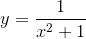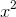Search IntMath
Close

450+ Math Lessons written by Math Professors and Teachers

5 Million+ Students Helped Each Year

1200+ Articles Written by Math Educators and Enthusiasts

Simplifying and Teaching Math for Over 23 Years

# Sampling to create mathematical function graphs

By Murray Bourne, 23 Sep 2015

## Sampling Functions

Graph sketching is a vital mathematical skill, whether we have access to computers or not. When modelling a data set, it's very useful to have a variety of curve shapes to choose from.

Curve sketching questions usually say something like:

"Sketch the given function, indicating main features of the graph."

When first learning to draw such function curves, students are taught to complete a table of values, something like this:

 x −3 −2 −1 0 1 2 3 y −2 −4 −5 0 2 4 6

As long as the graph is a "nice", well-behaved curve in the region −3 < x < 3, then drawing a table is not a bad starting point.

However, a lot of students continue to do this for more complicated functions, and end up in a lot of trouble. It's important to have a general idea what the curve will look like before you start, so you don't miss key attributes of the curve.

Whenever you see a graph of a function drawn on a computer, it involves sampling. Sampling is constrained in that you want to produce a smooth curve, but not have a large file size (or require a lot of computer processing time). When humans sketch a mathematical function curve, some of the same considerations need to be kept in mind.

Let's look at some examples.

### A symmetric curve

In this example (I'll tell you a bit later what the function is), we've sampled 4 x-values, completed the table, put the dots on the graph and joined them with straight lines.

 x −3 −1 1 3 y 0.1 0.5 0.5 0.1

We could have been a bit smarter and joined them with a curve, something like this. What do you think the function for this curve is?

I've seen this kind of sketch many times. It looks reasonable, but it's wrong. The student has not sampled enough points and the curve does not capture all the required characteristics.

The student remembers that we should sample some more points to get a more accurate curve. So we try 7 points this time. The spacing between each point is 1.5 units.

Now our curve is taking on quite a different shape. We don't know if y = 1 is the highest point, but we have a better curve than our first attempt.

The next example uses 50 sample points, and we can finally get a good idea of the final shape from this.

The curve we've been trying to draw is actually.

The maximum point is indeed (0, 1) and by considering what happens when x approaches negative infinity (on the left) and positive infinity (on the right), the denominator of the fraction becomes very large, so the fraction value becomes very small, and approaches 0.

Our first attempt indicated the curve passed through the x-axis, but we can see now that this is incorrect.

With enough sample points, we have captured all the required details on the graph. We can see the maximum point, and we can see the x-axis is an asymptote for the graph.

Very few students will draw up a table with 50 values! It's much better to examine the function first, and to consider what happens at some key points (like x = 0), and also what happens when x is very small, and very large. Another hint for drawing this function is that it's going to be symmetric about the y-axis, because of theterm in the denominator.

### A polynomial

It's a good idea to know the general shapes of quadratic, cubic, quartic and quintic curves. If you know in advance the likely shape of your polynomial curve, it's easier to draw with less data points, and you're more likely to include all the key features.

Let's look at an example where things can go wrong.

We are given a polynomial function and asked to draw it. We find some typical values, using 10 sample points.

It seems to be a fairly well-behaved curve. We confidently join the data points with a smooth curve, then move on to the next question.

But wait! Here's the original question:

"Sketch y = x4 + 8x3 − 13x2 − 92x + 96".

This is a quartic (highest power is 4), and this should alert us that there will be 2 arms of the curve that go off to positive infinity. However, our curve has only one arm going off to positive infinity, and the other going to negative infinity (or so it seems). There must be something wrong.

Let's try again with a wider view (we increase the range of x-values on the x-axis).

That's better! Our curve shows the 2 arms that go off to positive infinity (it's positive because the number in front of the x4 term is positive). There's no need to zoom out any further since we can now see the main features of the graph. (The x- and y-intercepts, the local maxima and minima, and the behavior as the x becomes very small and very large.)

### More complicated curve

Here's one that catches a lot of students. If you take the "plug values into a table" approach, you can get a really messy graph.

Our first try uses values that are about 0.5 units apart.

Once again, we draw a smooth curve through our points:

But it's not the correct graph. It has some of the right features (it suggests some repetition is going on and that it passes through (0, 0)), but it doesn't capture the key attributes.

Let's try again, this time sampling 70 points. Surely that should be enough?

This time it's still not good. We have a large gap between the first and second arms (what does that gap mean?), and a jagged join between the second and third arms.

Let's have another go with 250 points.

We can now see what the curve is meant to look like. It's actually the curve of the tangent function,

y = tan(x).

The problem with most graphing software is you often need to fiddle around like this to get a complete curve, and avoid unwanted joins.

In my svgPHPGrapher, I've built in the option to easily plot a curve where the slope is quite high, even when approaching a vertical line.

### Plotting the Inverse for a better curve

My approach in the next plot was to graph the inverse function

x = arctan(y)

This curve is mostly flat when looked at from the side (that is, turning the axes 90°, which is what an inverse function is all about).

This gives just one arm, as follows. It only needs around 35 sample points to produce a smooth curve.

Next, I repeat that arm across the board. I also inserted vertical asymtotes (the dashed lines) to indicate the curve gets closer and closer to those lines, but never touches them.

## Conclusion

While the approach of "plug values into the function, create a table, plot the points and then join them" is a reasonable place to start when teaching curve plotting, I feel it's important students should learn basic shapes of graphs. They are then less likely to have problems when sketching the curves by hand, and also when trying to use computer graphics software to draw curves.

### 3 Comments on “Sampling to create mathematical function graphs”

1. Alan Cooper says:

This is a very interesting topic. Not just from the point of view of graphing a given function but also from that of trying to guess the function that describes "what's really going on" behind experimental data.

In many sciences (especially social sciences) it is popular to construct a "mathematical model" without any theoretical basis. The problem with this is that there may be many such "models" which work equally well for the given data but are lousy at predicting new values. Unfortunately people are easily impressed by authoritative sounding references to a "mathematical" formula and so take predictions seriously when they should not.(And as a case in point, I think some of the situations people model with "hyperbolic discounting" are probably better explained with other kinds of functions.)

2. Rene Grothmann says:

Everything true, indeed. But thinking is harder than doing. So they do, type the values into a handheld and insert the results into the graph. Or even easier, type the function and press the plot button. That's okay for me. But we got to bring them into the thinking approach again.

3. Murray says:

### Comment Preview

HTML: You can use simple tags like <b>, <a href="...">, etc.

To enter math, you can can either:

1. Use simple calculator-like input in the following format (surround your math in backticks, or qq on tablet or phone):
a^2 = sqrt(b^2 + c^2)
(See more on ASCIIMath syntax); or
2. Use simple LaTeX in the following format. Surround your math with $$ and $$.
$$\int g dx = \sqrt{\frac{a}{b}}$$
(This is standard simple LaTeX.)

NOTE: You can mix both types of math entry in your comment.